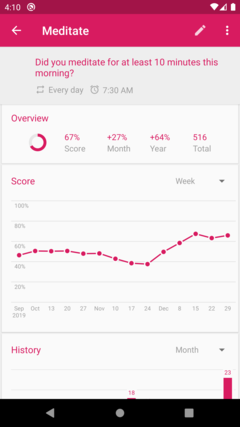# Habit Score

For long, I’ve used the Habit Tracker to keep track of my daily routines. A while ago, I’ve decided to stop using my Smartphone to reduce distracting. Instead, the task warrior has become my tool to keep track of daily routines. This comes with some downsides. I do not have that neat graphs anymore.To compensated, I’ve taken some time to figure out how the App is rendering the graph. After some search, I think I manage to find the formula that is used to compute the score value of a given habit. When we know this value, we can be used to generate an own graph.

$$s = 0.5^{\frac{\sqrt(f)}{13}}$$

Wait, what? $$0.5^{\frac{\sqrt{f}}{13}}$$ ? That’s an odd function. I have to admit, it took some time until I understood what the idea is. This is a graceful descending graph. The function will hit zero eventually. Important is the follow quote here:

    * Given the frequency of the habit, the previous score, and the value of
* the current checkmark, computes the current score for the habit.
*
* The frequency of the habit is the number of repetitions divided by the
* length of the interval. For example, a habit that should be repeated 3
* times in 8 days has frequency 3.0 / 8.0 = 0.375.
*/


A little bit below we can see the computation that is made:

        val multiplier = Math.pow(0.5, sqrt(frequency) / 13.0)
var score = previousScore * multiplier
score += checkmarkValue * (1 - multiplier)
return score


I wasn’t able to find any specify documentation within the project why this formula is the way it is. Habits require a certain amount of reiteration before it becomes a true habit. It might be that one of the paper that state this has made up this formula and the developer has adapted it. It could be also possible, that it was completely invented by him? I’m not certain.

How I understood the code is as follows: You compute for a fixed sized interval the number of repetitions. How often should you do this giving habit per week? In the graph on top of the page, we see the different interval lengths that available. There are fixed for weeks, months or years, when I remembered correctly. In the example the interval is 8 days what feels arbitrary, but it is just an example. Anyway, we then have the frequency.

At this point I’m a bit uncertain in how the app counts the actual number of repetitions. It could be that the frequency is a fixed value and the app computed via the value for a checkmark. Or it, changes frequency by calculate the actual repetitions one has done per interval into. It makes more sense to me that it is mainly done via the checkmark value because it is boolean.

One thing that left me to wonder is the 13? Why 13? I guess to represent the period something take until it becomes really a habit. When you remove it, it takes five days. I wrote the code in python to imitate it, but left the 13 as a factor away.

def score(frequency,previousScore, Value):
multipler = math.pow(0.5, math.sqrt(frequency))
score = previousScore * multipler
score += Value * (1 - multipler)
return score


Now we loop through and see:

It is day 0
Score is 0.5
It is day 1
Score is 0.75
It is day 2
Score is 0.875
It is day 3
Score is 0.9375
It is day 4
Score is 0.96875
It is day 5
Score is 0.984375


The formula converging against 1, but will never reach 1. This make sense because it represents percentage. Besides, when it has reached 0.99 it does not matter anyway, you would count it as 100%. We may keep playing with the code until we see the period in which a habit becomes habitual. This is fun because usually, we do not tinker with mathematical formula this way.

It also raises some concern here. Think about it, some people that use this app taking this as an indicated without understanding why it is this way. For them, it is just like: Hey here is a graph, and it looks great. Let this becomes a metric for this routine now. Yacs…

Browsing through the code base and I must say, Kotlin feels odd. However, this project is especially useful to learn from because it covers different platforms. I think that the code is organized as follows: A core part written in java to allow cross-platform support and then platform specify code. For instance, iOS or Android. It also has some web and server related resources. This make sense to keep the code arranged this way. I did just not expect this.

Any way, that it for now.

So far, akendo